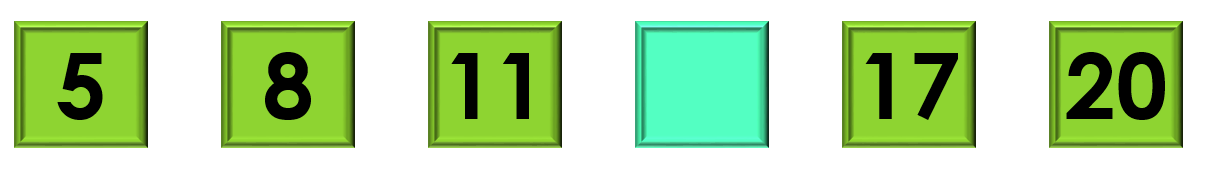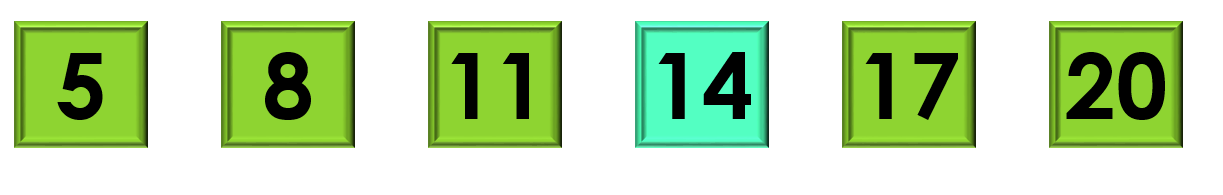1
visibility

Consider the given number pattern and identify the missing number.• A

15

• B

16

• C

14

• D

19

The correct answer is 14.

Solution:

Considering the given number pattern.Here, the numbers are increasing.
So, to find the missing number, we first find the rule.

Note that:
5 + 3 = 8
8 + 3 = 11
11 + 3 = 14
14 + 3 = 17
17 + 3 = 20

So, the rule is "add 3".Then, the missing number is 14.